International
Tables for
Crystallography
Volume F
Crystallography of biological macromolecules
Edited by M. G. Rossmann and E. Arnold

International Tables for Crystallography (2006). Vol. F, ch. 18.5, p. 403   | 1 | 2 |

## Section 18.5.1. Introduction

D. W. J. Cruickshanka*

aChemistry Department, UMIST, Manchester M60 1QD, England
Correspondence e-mail: dwj_cruickshank@email.msn.com

### 18.5.1. Introduction

| top | pdf |

#### 18.5.1.1. Background

| top | pdf |

Even in 1967 when the first few protein structures had been solved, it would have been hard to imagine a time when the best protein structures would be determined with a precision approaching that of small molecules. That time was reached during the 1990s. Consequently, the methods for the assessment of the precision of small molecules can be extended to good-quality protein structures.

The key idea is simply stated. At the conclusion and full convergence of a least-squares or equivalent refinement, the estimated variances and covariances of the parameters may be obtained through the inversion of the least-squares full matrix.

The inversion of the full matrix for a large protein is a gigantic computational task, but it is being accomplished in a rising number of cases. Alternatively, approximations may be sought. Often these can be no more than rough order-of-magnitude estimates. Some of these approximations are considered below.

Caveat. Quite apart from their large numbers of atoms, protein structures show features differing from those of well ordered small-molecule structures. Protein crystals contain large amounts of solvent, much of it not well ordered. Parts of the protein chain may be floppy or disordered. All natural protein crystals are noncentrosymmetric, hence the simplifications of error assessment for centrosymmetric structures are inapplicable. The effects of incomplete modelling of disorder on phase angles, and thus on parameter errors, are not addressed explicitly in the following analysis. Nor does this analysis address the quite different problem of possible gross errors or misplacements in a structure, other than by their indication through high B values or high coordinate standard uncertainties. These various difficulties are, of course, reflected in the values of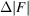used in the precision estimates.

On the problems of structure validation see Part 21of this volume and Dodson (1998).

Some structure determinations do make a first-order correction for the effects of disordered solvent on phase angles by application of Babinet's principle of complementarity (Langridge et al., 1960; Moews & Kretsinger, 1975; Tronrud, 1997). Babinet's principle follows from the fact that ifis constant throughout the cell, then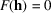, except for F(0). Consequently, if the cell is divided into two regions C and D,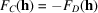. Thus if D is a region of disordered solvent,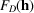can be estimated from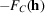. A first approximation to a disordered model may be obtained by placing negative point-atoms with very high Debye B values at all the ordered sites in region C. This procedure provides some correction for very low resolution planes. Alternatively, corrections are sometimes made by a mask bulk solvent model (Jiang & Brünger, 1994).

The application of restraints in protein refinement does not affect the key idea about the method of error estimation. A simple model for restrained refinement is analysed in Section 18.5.3, and the effect of restraints is discussed in Section 18.5.4and later.

Much of the material in this chapter is drawn from a Topical Review published in Acta Crystallographica, Section D (Cruickshank, 1999).

Protein structures exhibiting noncrystallographic symmetry are not considered in this chapter.

#### 18.5.1.2. Accuracy and precision

| top | pdf |

A distinction should be made between the terms accuracy and precision. A single measurement of the magnitude of a quantity differs by error from its unknown true value λ. In statistical theory (Cruickshank, 1959), the fundamental supposition made about errors is that, for a given experimental procedure, the possible results of an experiment define the probability density function f(x) of a random variable. Both the true value λ and the probability density f(x) are unknown. The problem of assessing the accuracy of a measurement is thus the double problem of estimating f(x) and of assuming a relation between f(x) and λ.

Precision relates to the function f(x) and its spread.

The problem of what relationship to assume between f(x) and the true value λ is more subtle, involving particularly the question of systematic errors. The usual procedure, after correcting for known systematic errors, is to suppose that some typical property of f(x), often the mean, is the value of λ. No repetition of the same experiment will ever reveal the systematic errors, so statistical estimates of precision take into account only random errors. Empirically, systematic errors can be detected only by remeasuring the quantity with a different technique.

Care is needed in reading older papers. The word accuracy was sometimes intended to cover both random and systematic errors, or it may cover only random errors in the above sense of precision (known systematic errors having been corrected).

In recent years, the well established term estimated standard deviation (e.s.d.) has been replaced by the term standard uncertainty (s.u.). (See Section 18.5.2.3on statistical descriptors.)

#### 18.5.1.3. Effect of atomic displacement parameters (or temperature factors')

| top | pdf |

It is useful to begin with a reminder that the Debye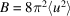, where u is the atomic displacement parameter. If B = 80 Å2, the r.m.s. amplitude is 1.01 Å. The centroid of an atom with such a B is unlikely to be precisely determined. For B = 40 Å2, the 0.71 Å r.m.s. amplitude of an atom is approximately half a C—N bond length. For B = 20 Å2, the amplitude is 0.50 Å. Even for B = 5 Å2, the amplitude is 0.25 Å. The size of the atomic displacement amplitudes should always be borne in mind when considering the precision of the position of the centroid of an atom.

Scattering power depends on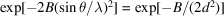. For B = 20 Å2 and d = 4, 2 or 1 Å, this factor is 0.54, 0.08 or 0.0001. For d = 2 Å and B = 5, 20 or 80 Å2, the factor is again 0.54, 0.08 or 0.0001. The scattering power of an atom thus depends very strongly on B and on the resolution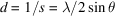. Scattering at high resolution (low d) is dominated by atoms with low B.

An immediate consequence of the strong dependence of scattering power on B is that the standard uncertainties of atomic coordinates also depend very strongly on B, especially between atoms of different B within the same structure.

[An IUCr Subcommittee on Atomic Displacement Parameter Nomenclature (Trueblood et al., 1996) has recommended that the phrase temperature factor', though widely used in the past, should be avoided on account of several ambiguities in its meaning and usage. The Subcommittee also discourages the use of B and the anisotropic tensor B in favour of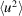and U, on the grounds that the latter have a more direct physical significance. The present author concurs (Cruickshank, 1956, 1965). However, as the use of B oris currently so widespread in biomolecular crystallography, this chapter has been written in terms of B.]

### References

Cruickshank, D. W. J. (1956). The determination of the anisotropic thermal motion of atoms in crystals. Acta Cryst. 9, 747–753.Google Scholar
Cruickshank, D. W. J. (1959). Statistics. In International tables for X-ray crystallography, Vol. 2, edited by J. S. Kasper & K. Lonsdale, pp. 84–98. Birmingham: Kynoch Press.Google Scholar
Cruickshank, D. W. J. (1965). Notes for authors; anisotropic parameters. Acta Cryst. 19, 153.Google Scholar
Cruickshank, D. W. J. (1999). Remarks about protein structure precision. Acta Cryst. D55, 583–601.Google Scholar
Dodson, E. (1998). The role of validation in macromolecular crystallography. Acta Cryst. D54, 1109–1118.Google Scholar
Jiang, J.-S. & Brünger, A. T. (1994). Protein hydration observed by X-ray diffraction. Solvation properties of penicillopepsin and neuraminidase crystal structures. J. Mol. Biol. 243, 100–115.Google Scholar
Langridge, R., Marvin, D. A., Seeds, W. E., Wilson, H. R., Hooper, C. W., Wilkins, M. H. F. & Hamilton, L. D. (1960). The molecular configuration of deoxyribonucleic acid. II. Molecular models and their Fourier transforms. J. Mol. Biol. 2, 38–64.Google Scholar
Moews, P. C. & Kretsinger, R. H. (1995). Refinement of the structure of carp muscle calcium-binding parvalbumin by model building and difference Fourier analysis. J. Mol. Biol. 91, 201–228.Google Scholar
Tronrud, D. E. (1997). TNT refinement package. Methods Enzymol. 277, 306–319.Google Scholar
Trueblood, K. N., Bürgi, H.-B., Burzlaff, H., Dunitz, J. D., Gramaccioli, C. M., Schulz, H. H., Shmueli, U. & Abrahams, S. C. (1996). Atomic displacement parameter nomenclature. Report of a subcommittee on atomic displacement parameter nomenclature. Acta Cryst. A52, 770–781.Google Scholar